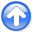First-break traveltime tomography with the double-square-root eikonal equationNext: Theory Up: Li et al.: DSR Previous: Li et al.: DSR

# Introduction

The first-break traveltime tomography (Osypov, 2000; Noble et al., 2010; Zhu et al., 1992; Leung and Qian, 2006; Taillandier et al., 2009) has been an established tool for estimating near-surface macro-feature seismic velocities. Starting from a prior model, tomographic inversion gradually modifies the velocities such that the misfits between predicted and observed first-breaks decrease. Since the problem is nonlinear, several linearization iterations may be required until convergence. Moreover, inversion must be carried out with careful choice of regularization in order to avoid local minima (Simmons and Bernitsas, 1994; Stefani, 1993; Engl et al., 1996). The estimated model has a direct influence on subsequent applications, for example static corrections (Marsden, 1993; Bergman et al., 2004; Cox, 1999) where it provides a medium-to-long wavelength near-surface model, and waveform tomography (Sheng et al., 2006; Virieux and Operto, 2009; Brenders and Pratt, 2007) where it serves as a low-frequency prior.

The traditional first-break traveltime tomography is based on the eikonal equation that arises from high-frequency approximation of the wave equation (Chapman, 2002). During forward modeling, the first-breaks computed through the eikonal equation are naturally shot-indexed because only receiver coordinates move while the source is fixed. At tomography stage, one may formulate the minimization of cost function as a sequence of explicitly linearized problems or directly as a nonlinear optimization problem. The first choice (Zelt and Barton, 1998; Dessa et al., 2004; Zhu et al., 2000; Pei, 2009) requires computation of Fréchet derivatives, which is usually carried out by combining an eikonal solver with posterior ray tracing. Then an algorithm such as LSQR (Paige and Saunders, 1982) is applied to solve the linearized tomographic system iteratively. While this approach accounts for information from both source and receiver dimensions, it faces computational limitations when the Fréchet derivative matrix becomes difficult to handle because of a large number of model parameters. The nonlinear optimization approach, on the other hand, can be combined with the adjoint-state method (Plessix, 2006) and avoids an explicit computation of Fréchet derivatives (Taillandier et al., 2009). The cost of computing gradient is equivalent to twice the solution of the forward modeling problem, regardless of the size of input data. However, one major drawback of this approach, as we will show later, is that the resulting gradient disregards information available along the shot dimension.

The drawback of eikonal-based adjoint-state tomographies is that they always face conflicting information that propagates across different shots. Such conflicts must be resolved during inversion, or else an erroneous model update may appear. In practice, the inversion may be less robust and may take more iterations to converge, compared to the situation where we replace the eikonal equation with another governing equation that allows both source and receiver positions to change along ray-paths. The double-square-root (DSR) eikonal equation is a promising candidate in this regard, because it describes the prestack data as a whole by linking the evolution of traveltimes to both sub-surface source and receiver positions. In this paper, we investigate the feasibility of using the DSR eikonal equation for first-break traveltime tomography with the adjoint-state method.

DSR eikonal was analyzed previously by Belonosova and Alekseev (1974), Duchkov and de Hoop (2010) and Alkhalifah (2011). Ray-tracing methods applied to DSR are capable of providing multi-arrivals by extrapolating isochron rays (Iversen, 2004) or using perturbation theory, but their extra costs in computing non-first-breaks are not necessary for first-break tomography purpose. We first prove that an implicit discretization of the DSR eikonal equation is causal and thus can be solved by a Dijkstra-like non-iterative method (Dijkstra, 1959). The DSR singularity and two DSR branches that are non-causal need special treatment. Our current implementation employs a modified fast-marching (Sethian, 1999) DSR eikonal solver. We first test its accuracy by DSR forward modeling. Next, we linearize the DSR eikonal equation and use the resulting operators in adjoint-state tomography. For comparison, we apply an analogous linearization and adjoint-state formulation to the traditional tomography based on shot-indexed eikonal equation. Then we demonstrate the differences between the proposed and traditional approaches and justify advantages of the new method using several synthetic model examples. We conclude by discussing possible further improvements and extensions of our method.First-break traveltime tomography with the double-square-root eikonal equationNext: Theory Up: Li et al.: DSR Previous: Li et al.: DSR

2013-10-16